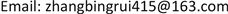1. 引言

2. 滑转率测算方法2.1. 系统设计

s = w R − v max ( w R , v ) × 100 % (1)

2.2. 算法设计

X ^ ( k | k − 1 ) = A × X ^ ( k − 1 | k − 1 ) (2)

P ( k | k − 1 ) = A × P ( k − 1 | k − 1 ) × A T + Q (3)

K g ( k ) = P ( k | k − 1 ) × H T × [ H × P ( k | k − 1 ) × H T + R ( k + 1 ) ] − 1 (4)

P ( k | k ) = [ I − K g ( k ) × H ] × P ( k | k − 1 ) (5)

{ X ( k + 1 ) = A × X ( k ) + W ( k ) Z ( k ) = H × X ( k ) + V ( k ) (7)

X ( k ) = [ v ( k )     a ( k )     w ( k )     a w ( k ) ] T

A = [ 1 d t 0 0 0 1 0 0 0 0 1 d t 0 0 0 1 ] , H 2 = [ 1 0 0 0 0 0 1 0 ]

3. 实测实验

3.1. 柏油路面下不同速度的测试情况

3.2. 不同路况的测试情况

4. 总结与展望

1) 同一路面状况下，速度越快，滑转率波动越小。速度稳定情况下，柏油路车滑转率波动较小，砂石路、草地滑转率波动较大。

2) 实测结果说明该方法测量的驱动轮滑转率客观准确地反映了车辆运动规律，消除了测量值中的有害噪声及扰动，结果具有动态实时性。